# GSEB Solutions Class 10 Maths Chapter 7 Coordinate Geometry Ex 7.2

Gujarat Board GSEB Solutions Class 10 Maths Chapter 7 Coordinate Geometry Ex 7.2 Textbook Questions and Answers.

## Gujarat Board Textbook Solutions Class 10 Maths Chapter 7 Coordinate Geometry Ex 7.2

Question 1.
Find the coordinates of the point which divides the line segment joining the points (-1, 7) and (4, -3) in the ratio 2: 3.
Solution:
Let the given points be A(-1, 7) and B(4, -3).
Here, we have
x1 = -1, y1 = 7
x2 = 4, y2 = -3
and m1 = 2, m2 = 3
Let the required point be P(x, y).
Then, x = $$\frac{m_{1} x_{2}+m_{2} x_{1}}{m_{1}+m_{2}}$$
and y = $$\frac{m_{1} y_{2}+m_{2} y_{1}}{m_{1}+m_{2}}$$
⇒ x = $$\frac{2(4)+3(-1)}{2+3}$$ = $$\frac{8-3}{5}$$ = $$\frac{5}{5}$$ = 1
And y = $$\frac{2(-3)+3(7)}{2+3}$$ = $$\frac{-6+21}{5}$$ = $$\frac{15}{5}$$ = 3
Therefore, the required point is (1, 3).Question 2.
Find the coordinates of the points of tri-section of the line segment joining (4, -1) and (-2, -3).
Solution:
Let P and Q be the points of the tri-section of AB.
Then,
AP = PQ = QB = 1
Case I: Here P divides AB in the ratio 1 : 2.
So, we have
x1 = 4, y1 = -1
x2 = -2, y1 = -3
and m1 = 1, m2 = 2
∴ The coordinates of P are given by
= p $$\left[\frac{m_{1} x_{2}+m_{2} x_{1}}{m_{1}+m_{2}}+\frac{m_{1} y_{2}+m_{2} y_{1}}{m_{1}+m_{2}}\right]$$
= p $$\left[\frac{1(-2)+2(4)}{1+2}, \frac{1(-3)+2(-1)}{1+2}\right]$$
= p $$\left[\frac{-2+8}{3}, \frac{-3-2}{3}\right]$$
= p $$\left[\frac{6}{3}, \frac{-5}{3}\right]$$
= p $$\left[\frac{6}{3}, \frac{-5}{3}\right]$$
= p $$\left[2, \frac{-5}{3}\right]$$Case II:
Here, Q divides AB in the ratio 2: 1.
So, we have
x1 = 4, y1 = -1
x2 = -2, y2 = -3
and m1 = 2, m2 = 1
∴ The coordinates of Q are given by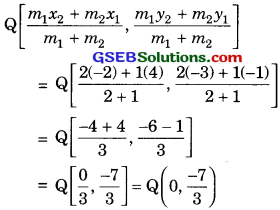∴ Coordinates of required points are $$\left[2, \frac{-5}{3}\right]$$ and $$\left[0, \frac{-7}{3}\right]$$.

Question 3.
To conduct Sports Day activities in your rectangular shaped school ground ABCD, lines have been drawn with chalk powder at a distance of 1 m each. 100 flower pots have been placed at a distance of 1 m from each other along AD, as shown in Figure. Niharika runs $$\frac {1}{4}$$th the distance AD on the 2 line and posts a green flag. Preet runs $$\frac {1}{5}$$th distance AD on the eighth line and posts a red flag. What is the distance between both the flags? If Rashmi has to post a blue flag exactly halfway between the line segment joining the two flags, where should she post her flag?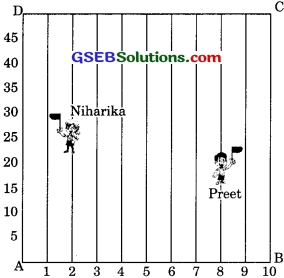Solution:
From the above figure, the position of given flag posted Niharika is M(2 $$\frac {1}{4}$$ x 100), i.e. M(2, 25)
and red flag post by Preet is N(8, $$\frac {1}{5}$$ x 100), i.e., N(8, 20).
Now, MN = $$\sqrt{(8-2)^{2}+(20-25)^{2}}$$
= $$\sqrt{6^{2}+(-5)^{2}}$$
= $$\sqrt{36+25}$$ = $$\sqrt{61}$$
Hence, the distance between the flags = $$\sqrt{61}$$ m and position of the blue flag post be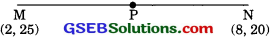P is given by = $$\left(\frac{x_{1}+x_{2}}{2}, \frac{y_{1}+y_{2}}{2}\right)$$
= $$\left(\frac{2+8}{2}, \frac{25+20}{2}\right)$$
= $$\left(\frac{10}{2}, \frac{45}{2}\right)$$ = (5, 22.5)
Hence, the blue flag is on the fifth line at a distance of 22.5 m above it.Question 4.
Find the ratio in which the segment joining the points (-3, 10) and (6, -8) is divided by (-1, 6).
Solution:
Let P(-1, 6) divides the line segment joining the points A(-3, 10) and B(6, -8) in the ratio k: 1.
Then the coordinates of P are given by –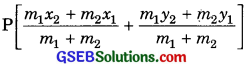Here, we have
x1 = -3, y1 = 10
x2 = 6, y2 = -8
and m1 = k, m2 = 1
So, the coordinates of P are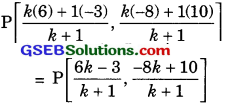But the coordinates of P are given as P(-1, 6)
∴ $$\frac{6 k-3}{k+1}$$ = -1
⇒ 6k -3 = -1(k + 1)
⇒ 6k -3 = -k – 1
⇒ 7k = 2
⇒ k = $$\frac{2}{7}$$
and = $$\frac{2}{7}$$ = 6
⇒ -8k + 10 = 6(k + 1)
⇒ -8k + 10 = 6k + 6
⇒ -8k – 6k = 6 – 10
⇒ -14k = -4
⇒ k = $$\frac{4}{14}$$ = $$\frac{2}{7}$$
Hence, required ratio = 2 : 7.Question 5.
Find the ratio in which the line segment joining A(1, -5) and B(-4, 5) is divided by the x-axis. Also find the coordinates of the point of divisions.
Solution:
Let the given points be A(1, -5) and B(-4, 5).
Let the x-axis cuts AB at the point P in the ratio k :1.
Then, the coordinates of P are given asHere, we have
x1 = 1, y1 = -5
x2 = -4 y2 = 5
and m1 = k, m1 = 1
So, coordinates of P are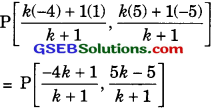Since, P lies on x-axis. So its ordinate is 0.
∴ $$\frac{5 k-5}{k+1}$$ = 0
⇒ 5k – 5 = 0
⇒ 5k = 5
⇒ k = 1
Hence, required ratio = (1 : 1).
Now, the coordinates of the point of division are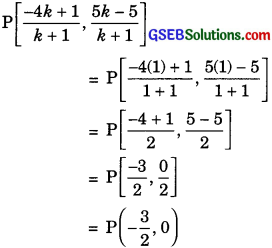Question 6.
If(1, 2), (4,y), (x, 6) and (3, 5) are the vertices of a parallelogram taken in order, find x and y.
Solution:
Let A(1, 2), B(4, y), C(x, 6) and D(3, 5) are the vertices of a parallelogram.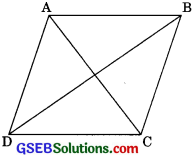We know that the diagonals of parallelogram bisect each other. So,
Coordinates of the mid-point of AC
= Coordinates of the mid-point of BD
⇒ $$\left(\frac{1+x}{2}, \frac{2+6}{2}\right)$$ = $$\left(\frac{4+3}{2}, \frac{y+5}{2}\right)$$
⇒ $$\left(\frac{1+x}{2}, 4\right)$$ = $$\left(\frac{7}{2}, \frac{y+5}{2}\right)$$
⇒ $$\frac {1 + x}{2}$$ = $$\frac {7}{2}$$
⇒ 1 + x = 7
⇒ x = 6
and y = $$\frac {y + 5}{2}$$
⇒ y + 5 = 8
⇒ y = 3
Hence, required values are x = 6 and y = 3.Question 7.
Find the coordinates of point A, where AB is the diameter of a circle whose center is (2, -3) and B is (1, 4).
Solution:
Let the given point be A(x, y). Since C is the mid-point of AB.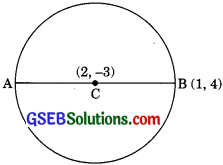∴ The coordinates of C are
= C $$\left[\frac{x_{1}+x_{2}}{2}, \frac{y_{1}+y_{2}}{2}\right]$$
= C $$\left[\frac{x+1}{2}, \frac{y+4}{2}\right]$$
But, the coordinates of C are (2, -3)
⇒ $$\frac {1 + x}{2}$$ = 2 and $$\frac {y + 4}{2}$$ = -3
⇒ x + 1 = 4 and y + 4 = -6
x = 4 – 1 and y = -6 -4
x = 3 and y = -10
Hence, the coordinates of A are (3, -10).Question 8.
If A and B are (-2, -2) and (2, -4) respectively, find the coordinates of P such that AP = AB and P lies on the line segment AB.
Solution:
AP = $$\frac {3}{7}$$AB
7AP = 3AB
7AP = 3(AP + PB)
[∴ p lines in the line segment AB]
7AP = 3AP + 3PB
4AP = 3PB
$$\frac {AP}{PB}$$ = $$\frac {3}{4}$$
We have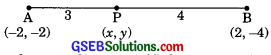Let the coordinates of P be (x, y). Then,
x = $$\frac{m_{1} x_{2}+m_{2} x_{1}}{m_{1}+m_{2}}$$
= $$\frac{(3)(2)+(4)(-2)}{3+4}$$
= $$\frac {6 – 8}{7}$$ = $$\frac {2}{7}$$

and y = $$\frac{m_{1} y_{2}+m_{2} y_{1}}{m_{1}+m_{2}}$$
= $$\frac{(3)(-4)+(4)(-2)}{3+4}$$
= $$\frac{-12-8}{7}$$ = –$$\frac{20}{7}$$
Hence, the coordinates of the point P be (-$$\frac{2}{7}$$,- $$\frac{20}{7}$$)Question 9.
Find the coordinates of the points which divide the line segment joining A(-2, 2) and B(2, 8) into four equal parts.
Solution:
Let P, Q and R be the three points that divide the line segment joi fling the points A(-2, 2) and (2, 8) in four equal parts.
Case I: For point P, we have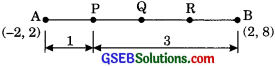Here, m1 = 1, m2 = 3
x1 = -2, y2 = 2
x2 = 2,y2 = 8
Then, coordinates of P are given by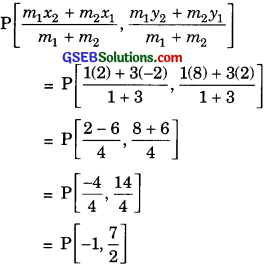Case II: For point Q, we have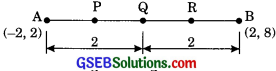m1 = 2, m2 = 2
x1 = -2, y2 = 2
and x1 = 2, y2 = 8
Then, coordinates of Q are given by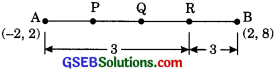= Q $$\frac{-12-8}{7}$$ = Q (0, 5)

Case III: For point R, we have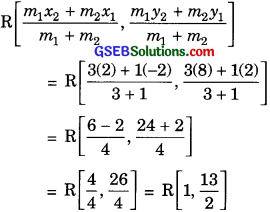Question 10.
Find the area of a rhombus if its vertices are (3, 0), (4, 5), (-1, 4) and (-2, -1) taken in order.
Solution:
Let the given points are A(3, 0), B(4, 5), C(-1, 4)
and D(-2, -1).
We have,
BD = $$\sqrt{(-2-4)^{2}+(-1-5)^{2}}$$
= $$\sqrt{(-6)^{2}+(-6)^{2}}$$
= $$\sqrt{36+36}$$ = $$\sqrt{72}$$

AC = $$\sqrt{(-1-3)^{2}+(4-0)^{2}}$$
= $$\sqrt{(-4)^{2}+(4)^{2}}$$
= $$\sqrt{16+16}$$ = $$\sqrt{32}$$
= 4$$\sqrt{2}$$
Now, area of rhombus ABCD
= $$\frac{1}{2}$$ x (Product of diagonals)
Area of rhombus = x (AC x BD)
= $$\left[\frac{1}{2} \times 4 \sqrt{2} \times 6 \sqrt{2}\right]$$ sq. units
= (2$$\sqrt{2}$$ x 6$$\sqrt{2}$$) sq. units
= 24 sq. units